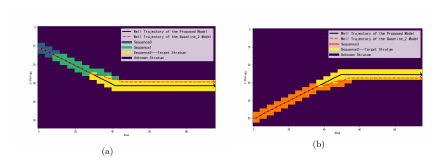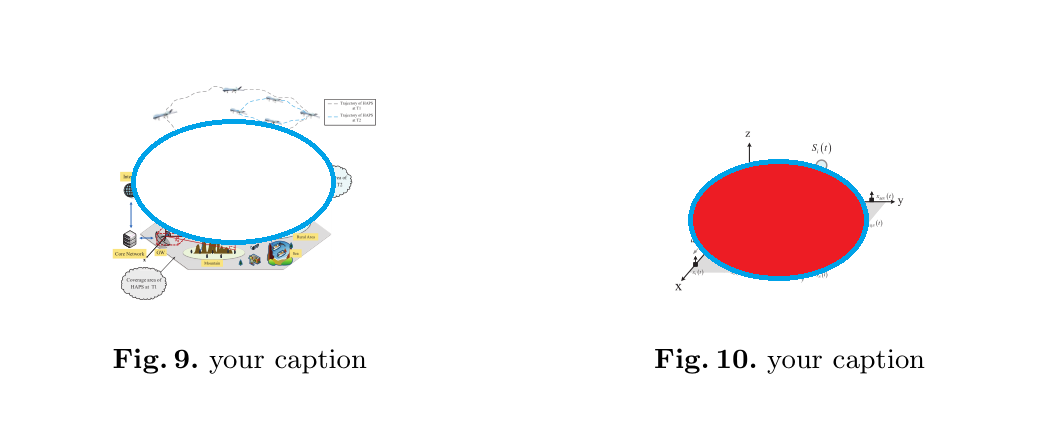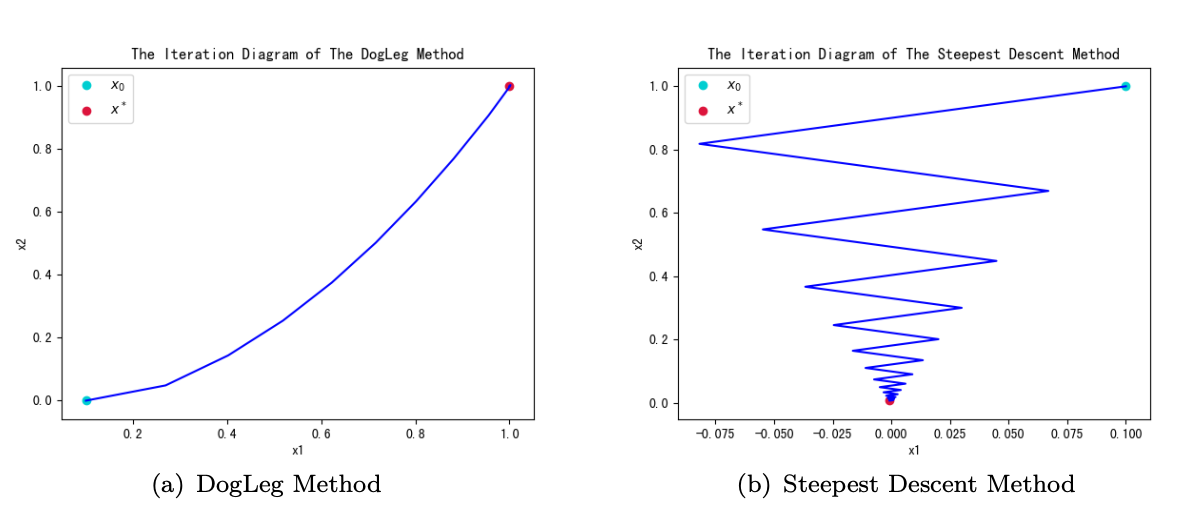• Latex两张图片并列显示

千次阅读 2018-12-29 15:01:46
Latex的编辑中经常会遇到使得两张图片并列显示的情况。 %\usepackage{caption} %\usepackage{subfigure} %导言区域要添加以上两个包 \begin{figure}[htbp] \centering \subfigure[] { \begin{minipage...

在Latex的编辑中经常会遇到使得两张图片并列显示的情况。

%\usepackage{caption}
%\usepackage{subfigure}
%导言区域要添加以上两个包

\begin{figure}[htbp]
\centering
\subfigure[]
{
\begin{minipage}{7cm}
\centering
\includegraphics[scale=0.27]{figs/fig14.png}
\end{minipage}
}
\subfigure[]
{
\begin{minipage}{7cm}
\centering
\includegraphics[scale=0.27]{figs/fig15.png}
\end{minipage}
}
\label{fig:14}
\end{figure}

实际效果图如图所示具体转自如何使得Latex中的图片并列展示？
谢谢大家！

展开全文Latex
• 两张图片并排显示 \begin{figure}[H] \centering \begin{minipage}{.5\textwidth} \centering \includegraphics[width=.8\linewidth]{images/logo1.png} \caption{Figure \#1} \label{fig:fig1} \end{minipage}%...

两张图片并排显示

\begin{figure}[H]
\centering
\begin{minipage}{.5\textwidth}
\centering
\includegraphics[width=.8\linewidth]{images/logo1.png}
\caption{Figure \#1}
\label{fig:fig1}
\end{minipage}%
\begin{minipage}{.5\textwidth}
\centering
\includegraphics[width=.8\linewidth]{images/logo2.png}
\caption{Figure \#2}
\label{fig:fig2}
\end{minipage}
\end{figure}

两张图片纵列显示，页码展示在左侧

\begin{figure}[H]
\centering
\includegraphics[scale=0.4]{images/chart.png}
\caption{Original Timeline}
\label{fig:chart}
\end{figure}

\begin{figure}[H]
\centering
\includegraphics[scale=0.4]{images/chart1.png}
\caption{Updated Timeline}
\label{fig:new_chart}
\end{figure}

展开全文latex
• Latex实现图片并列排放

千次阅读 2019-02-27 21:01:42
以下代码实现两张图片并列排放（单张图片拥有独立标题） \begin{figure}[h] \begin{minipage}[t]{0.5\linewidth} #0.5表示宽度可以调节 \centering \includegraphics[width=0.8\textwidth]{fig1.eps} ...

Latex实现图片并列排放

#首先导入包文件

\usepackage{subfigure}

以下代码实现两张图片的并列排放（单张图片拥有独立标题）

\begin{figure}[h]
\begin{minipage}[t]{0.5\linewidth}	#0.5表示宽度可以调节
\centering
\includegraphics[width=0.8\textwidth]{fig1.eps}	#fig1.eps是图片名
\end{minipage}
\hfill
\begin{minipage}[t]{0.5\linewidth}
\centering
\includegraphics[width=0.8\textwidth]{fig2.eps}
\end{minipage}
\end{figure}

最终效果如下图所示：如果有错误的地方还请大家多多谅解与指正==

展开全文latex latex图片并列 latex图片
• LaTeX两张图并排显示

千次阅读 2020-04-08 17:42:14
\usepackage{caption} % 图片脚注 \usepackage{graphicx} \usepackage{caption} \usepackage{subfigure} % 子图包 二、具体代码 \begin{figure}[htbp] \subfigure[DogLeg Method] %第一张子图 { \begin{...

一、需要引入的包

\usepackage{caption}   % 图片脚注
\usepackage{graphicx}
\usepackage{caption}
\usepackage{subfigure} % 子图包

二、具体代码

\begin{figure}[htbp]
\subfigure[DogLeg Method] %第一张子图
{
\begin{minipage}{6cm}
\centering          %子图居中
\includegraphics[scale=0.4]{dogleg.png}   %以pic.jpg的0.4倍大小输出
\end{minipage}
}
\subfigure[Steepest Descent Method] %第二张子图
{
\begin{minipage}{7cm}
\centering      %子图居中
\includegraphics[scale=0.4]{steepest.png}   %以pic.jpg的0.4倍大小输出
\end{minipage}
}
%\caption{name of the figure} %  %大图名称
%\label{fig:1}  %图片引用标记
\end{figure}切记\subfigure之外如果加了\centering两幅图就不会并列了，需要加在\subfigure之内。

展开全文latex
• 这里写目录标题）正常插入图片并列插入两张图片 正常插入图片 \begin{figure} \includegraphics[width=0.5\textwidth]{figures/tree.eps} \caption{TGT_GTG​.} \label{tree} \end{figure} 如上述代码所示，首先要有...latex
• 最近需要用latex插入多张图片，达到这么一个效果。 但是我原来只插入过一张图片图片内容来源于网络；是国漫一人之下的宝儿姐。强推这部国漫~），代码如下，效果如图： \begin{figure} \centering \...latex
• LaTeX如何让两张图并排显示？

万次阅读 多人点赞 2018-01-30 15:14:22
%\usepackage{caption} ...%导言区域要添加以上个包 \begin{figure}[htbp] \centering %居中 \subfigure[name of the subfigure] %第一张子图 { \begin{minipage}{7cm} \centering %子图居中 \inLaTeX
• 如题, 分享一个latex中将两张图片分别作为一个图, 并将这两张并列放置的源代码. 其效果图如下图所示: 其源代码如下: \documentclass{article} \usepackage{graphicx} \begin{document} \begin{figure} \...
•latex
• latex两个表格并列

万次阅读 2019-08-15 21:56:01
\\[12pt]%设置个表格之间的空白行距离 \begin{minipage}[!t]{\columnwidth} \renewcommand{\arraystretch}{1.3} \caption{表格2标题} \label{表格2标签} \centering \setlength{\tabcolsep}{0.8mm}{ \begin{...
• 有一个任务就是如何将四pdf（当然也可以是其他格式的）合并为一个文件，并且是行两列排列。 在网上找了半天的资料，很多代码跑不通，最后修改了一些内容，终于成功了~ ps：里面很多宏包并没有用到，因为我是...latex
• latex画两个并列的图

千次阅读 2020-02-14 09:36:31
\begin{figure}[t] \vspace{-0.1in} \captionsetup[subfigure]{skip = 0pt} \centering \subfloat[Partition Overview]{\includegraphics[width = 1.5in]{fig/partition.eps}\label{fig:partition...latex
• \begin{figure}[H] \centering %居中 \subfigure[name of ...{102.png} %以pic.jpg的0.5倍大小输出 \end{minipage} } \caption{name of the figure} % %大图名称 \label{fig:1} %图片引用标记 \end{figure}latex
• Latex】【插入图片】如何在latex中插入并列图片 实验分析部分需要将多个图片并列展示，今天看了网友的一些提示，写出了种并列展示图片的方法： 每个图片都有一个标题 多个图片共用一个标题，每个图片有自己的...latex
• 一、一行多列图片排版 二、一列多行图片排版 三、多列多行图片排版 四、双列多行图片排版 在文献撰写过程中，有时需要进行多图片的比较，下面我就分享下我的解决办法 下面直接上代码，代码的大致思路是，利用\...latex
• Latex多图并列

2020-03-29 10:22:07
代码如下： \begin{figure}[tb] % \centering % \subfigure{ \begin{minipage}[h]{0.33\linewidth} \centering \...}% % \vspace{2mm} \caption{整个两张图的解说} \label{fig:tsne} \end{figure}latex
• latex插入并列图片

千次阅读 2017-05-30 17:20:16
插入并列图片需要使用到的宏： \usepackage{graphicx} \usepackage{subfigure} \begin{figure}[h] \begin{minipage}[t]{0.4\linewidth} \centering \includegraphics[width=1.2\textwidth]{Fig3.pdf} \captiolatex
• latex--多个图片并排

万次阅读 2019-06-05 20:22:35
latex中插入图片是非常常见的操作，这里给出插入一个图片的做法和相应的代码。 %制作者：ruikunw2015@126.com %编译方式：latex \documentclass{article} \usepackage{ctex} \usepackage{graphicx}%图片头文件 \...latex图片插入
• LaTeX如何让两张图并排显示

千次阅读 2020-03-28 15:47:49
转载 ...%\usepackage{caption} %\usepackage{subfigure} %导言区域要添加以上个包 \begin{figure}[htbp] \centering %居中 \subfigure[name o...
• 感谢博主的启发，使用了该博主提供的代码，的确可以实现两张表格并排，但是有个问题就是，我加入了\caption{表格注释} 总是失败，排查了一下，发现原因是因为这位博主使用的代码块是\begin{minipage}，这个代码块是...latex
• 这篇文章中介绍了使用minipage的方式混排图片和文字，达到图片在右侧，文字在左侧的效果。 注意minipage可以当做一个page来使用，它独立成为一个单元，里面可以添加figure, tabular, equation, text等资源。 例如...linux latex 语法
• 关于Latex并排两张表格的解决方案

万次阅读 多人点赞 2017-11-27 16:34:34
今天在修改latex时，发现两张表格无论怎么修改还是一上一下，网络上有很多方法，但是效果不是特别好，可能是不同的环境会有不同的效果。 这篇博客呢，想给碰到同样问题的同仁一个可供参考的，我自己可行的方案，能...latex 解决方案 表格
• \begin{document}\begin{figure}[htbp]\begin{subfigure}[t]{0.3\textwidth}\includegraphics[width=\linewidth]{Images/1.png}\caption{}\end{subfigure}\begin{subfigure}[t]{0.3\textwidth}\includegraphics[widt...
• 使用latex的subfigure排版并排放置的两张图. \begin{figure} \begin{minipage}[t]{0.5\linewidth} \centering \includegraphics[width=2.2in]{fig1.eps} \caption{fig1} \label{fig:side:a} \...
• 一、图并列2*2排版：（外的1*2都可以参考下列代码） \begin{figure}[htbp] \centering \begin{minipage}{0.49\linewidth}%表示图片的占用那一列的宽度 \centering \vspace{-0.6cm}%表示图片与最上方的文字...latex
• 你使用 如下 latex \begin{figure}[h] \subfloat[]{ \label{picd} \includegraphics[width=6.77cm]{picd.pdf} } \subfloat[]{ \label{pice} \includegraphics[width=7.04cm]{pice.pdf} } \caption{$2m\times 2m$...latex微信 新浪微博
• 一，效果图：  二，代码： \begin{figure}[t] \centering  \begin{tabular}{cc} \begin{minipage}[t]{2in} \includegraphics[width=1.8in]{gestalt_theory/similarity.jpg} \caption{Similarit...
• Overleaf / Latex 中并排插入两张图片 最近在用overleaf写论文，遇到了需要并排插入两张图片的情况，在网上查了好久终于成功了，记录一下。 首先要上传你要插入的两个图片，点这个upload按钮，我上传了111.png和222....
• LaTeX 中使两张表格并排

千次阅读 2019-01-26 16:10:00
在使用 LaTeX写论文或者画海报的时候，希望两张较小的表格可以并排，（一般情况的LaTeX插入两张图片是上下布局的） 查找了一下，相关的例子如下： \begin{minipage}{\textwidth} \begin{minipage}[t]{0.45\......